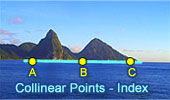# Collinear Points, Theorems and Problems - Table of Content 5Geometry Problem 678.
Triangle, Simson Line, Circumcircle, Tangent, Parallel, Perpendicular, Collinear Points.

Geometry Problem 675.
Intersecting Circles, Secant, 45 and 90 Degrees, Collinear Points.

Geometry Problem 669.
Triangle, Circumcircle, Incenter, Midpoint, Collinear points, Mind Map.

Geometry Problem 659.
Parallelogram, Parallel Lines, Collinear Points.

Geometry Problem 632.
Triangle, Interior and Exterior Angle Bisectors, Midpoints, Collinear Points.

Go to page:  Previous | 1 | 2 | 3 | 4 | 5 | 6 | 7 | 8 | 9 | 10 | Next